# Using Sum on List ItemsHi,

In one of our models w have weeks as a list item, with approximately 76 weeks in total. In one module, each week has a line item 'Score'. In a separate module I'm trying to calculate the sum of score in a 4 week period (between weeks 31 and weeks 35. How would I go about doing this?

Thanks

Tagged:

•Assuming I have read it correctly that weeks is a list and not native time, if the list was created in the correct order, then it is a little know fact that you can use CUMULATE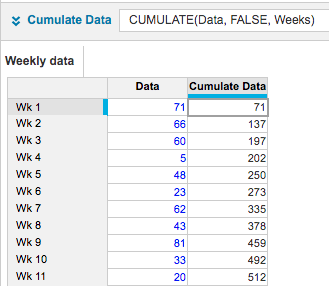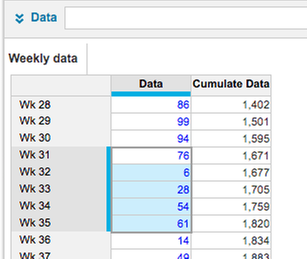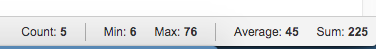You can then look for the final week and then subtract the start week.  If the below I've kept it really simple and entered the start after.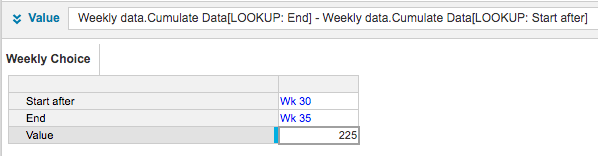If you want the entry to be week 31, then set up a module that holds the mappings and then use that to calculate the "start after" week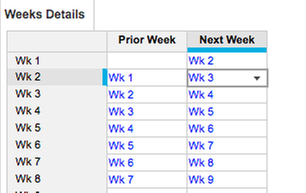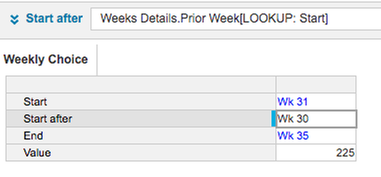I hope that helps

David